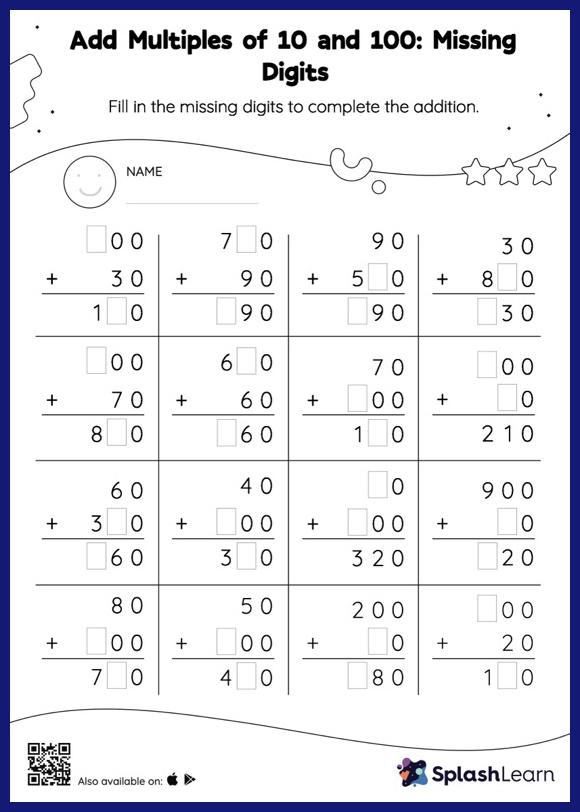# Add Multiples of 10 and 100: Missing Digits Worksheet

Home > Add Multiples of 10 and 100: Missing DigitsIf the missing number is an addend, students use the count on strategy or the relationship between addition and subtraction to find the missing number. And if the missing number is the sum of the addition sentence, they add the addends to get the sum. This worksheet, add multiples of 10 and 100, provides plenty of practice with this concept. As the worksheet uses the column method, it is helpful in getting students toward higher accuracy, especially with bigger numbers and in scenarios where regrouping is required.